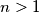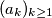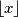### IMO Shortlist 2014 problem N4

Kvaliteta:
Avg: 0,0
Težina:
Avg: 7,0

Let$n > 1$ be a given integer. Prove that infinitely many terms of the sequence$(a_k)_{k \geq 1}$, defined byare odd. (For a real number$x$,$\lfloor x \rfloor$ denotes the largest integer not exceeding$x$.)

(Hong Kong)

Izvor: https://www.imo-official.org/problems/IMO2014SL.pdf